Joins allow you to combine data from two tables, using two steps:

• determining matches in values between a set of specified columns.
• merging columns from matching rows into a new table.

The number of ways joins can be used is surprisingly deep, and provides an important foundation for most data analyses!

``````from siuba import (
_,
inner_join, left_join, full_join, right_join,
semi_join, anti_join
)

lhs = pd.DataFrame({'id': [1,2,3], 'val': ['lhs.1', 'lhs.2', 'lhs.3']})
rhs = pd.DataFrame({'id': [1,2,4], 'val': ['rhs.1', 'rhs.2', 'rhs.3']})``````
``lhs``
id val
0 1 lhs.1
1 2 lhs.2
2 3 lhs.3
``rhs``
id val
0 1 rhs.1
1 2 rhs.2
2 4 rhs.3
Note

The format of graphs and the structure of examples takes heavy inspiration from R for Data Science.

## Syntax

Like other siuba verbs, joins can be used in two ways: by directly passing both data as arguments, or by piping.

``````# directly passing data
inner_join(lhs, rhs, on="id")

# piping
lhs >> inner_join(_, rhs, on="id")``````
id val_x val_y
0 1 lhs.1 rhs.1
1 2 lhs.2 rhs.2

Note that when used in a pipe, the first argument to the join must be `_`, to represent the data.

## How joins work

This page will use graphs to illustrate how joins work. They show the values being joined on for the left-hand side on the rows, and those for the right-hand side on the columns.

For example, consider the inner join from the last section:

``inner_join(lhs, rhs, on="id")``
id val_x val_y
0 1 lhs.1 rhs.1
1 2 lhs.2 rhs.2

Here is a graph for this inner join: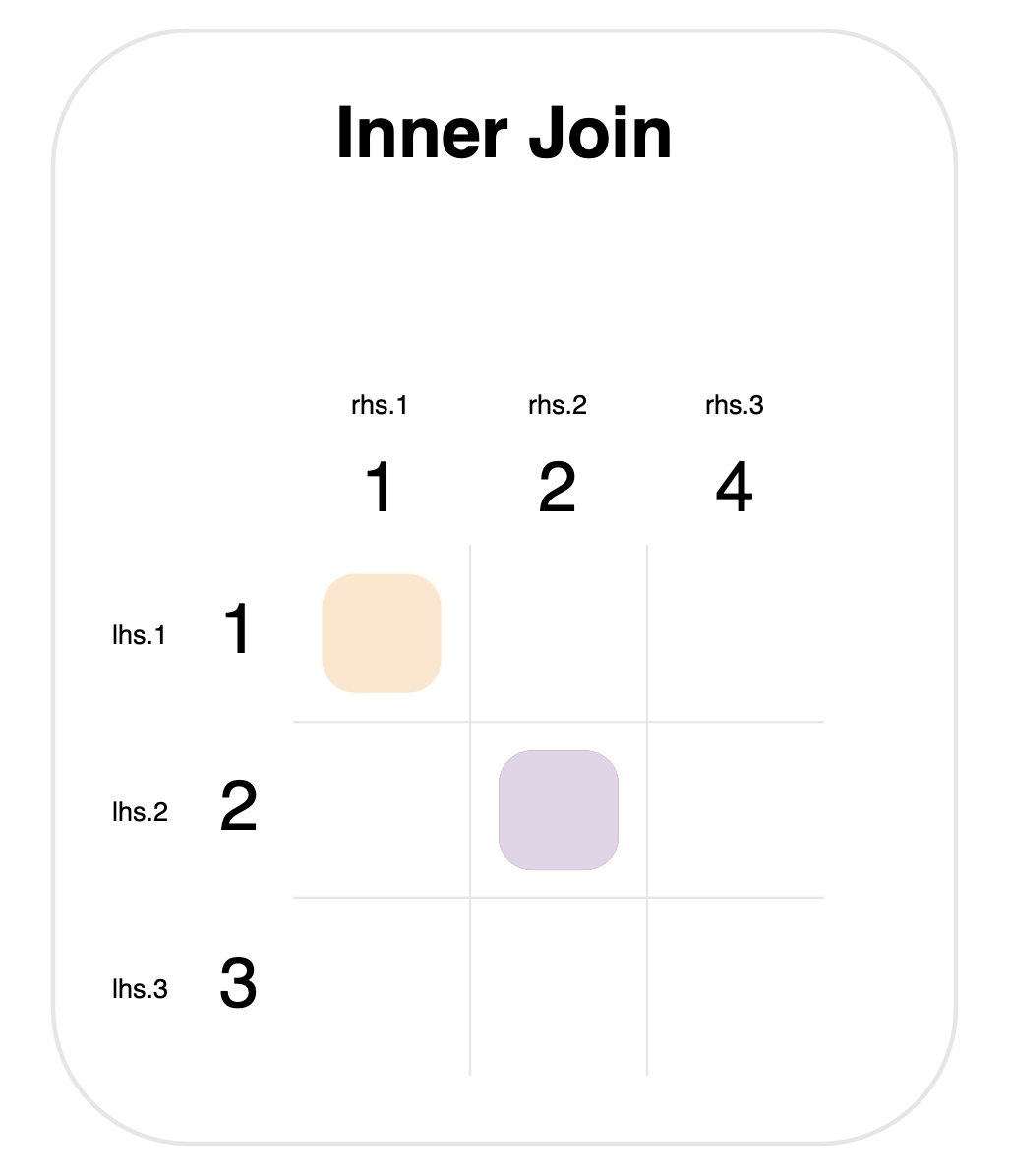Notice that `1, 2, 3` come from the `lhs.id` column, and `1, 2, 4` from `rhs.id`.

There are two steps to any join:

• Determine matches. The colored squares mark new rows that will be created. These are determined by looking at every pair of values from the left- and right-hand sides, and checking whether they’re equal. This means that there are 9 checks in total (one for each square in the grid).
• Merge rows. For each match, a row is created that has columns from both tables.

The next two sections on this page discuss different types of joins:

• Mutating joins: what should happen if a row or column doesn’t have any matches?
• Filtering joins: using matching rules without merging to filter the left-hand table.

The remaining four sections discuss important situations related to matching: duplicates, missing values, using multiple columns, and expressions.

## Mutating joins

Up to this point we’ve looked at an inner join. We’ll call this a mutating join, because it puts the columns from both tables into the final result. In this section, we’ll look at two more important joins: left join and full join.

The diagram below shows these three mutating joins.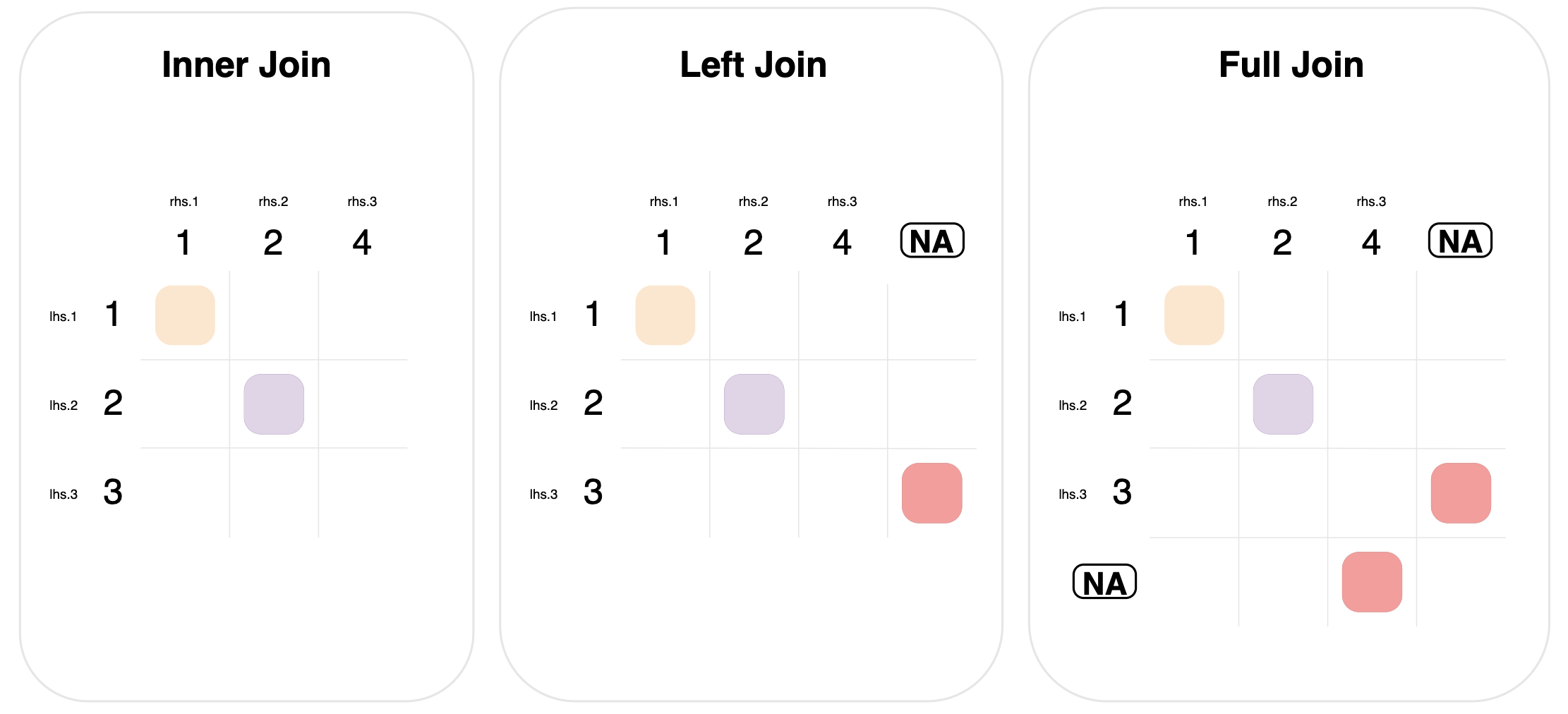Left and full joins define a new matching rule for rows of data that don’t have any matches:

Left join. Include rows from the left-hand table that didn’t have any matches. This is done by matching left-hand rows with no matches, to a “dummy” right-hand row of all missing values. This is signified in the graph by the circled NA column.

Full join: Similar to left join, but extended to include unmatched rows from both tables. Notice that the graph for full join has two “dummy circled NA” entries, one to match rows, and one to match columns.

### Inner join

``inner_join(lhs, rhs, on="id")``
id val_x val_y
0 1 lhs.1 rhs.1
1 2 lhs.2 rhs.2

### Outer joins

``left_join(lhs, rhs, on="id")``
id val_x val_y
0 1 lhs.1 rhs.1
1 2 lhs.2 rhs.2
2 3 lhs.3 NaN
``full_join(lhs, rhs, on="id")``
id val_x val_y
0 1 lhs.1 rhs.1
1 2 lhs.2 rhs.2
2 3 lhs.3 NaN
3 4 NaN rhs.3

## Filtering joins

Filtering joins use some of the same logic to determine matches as a join, but only to filter (remove) rows from the left-hand table.

We’ll use the data from the previous section to look at filtering joins.

``lhs``
id val
0 1 lhs.1
1 2 lhs.2
2 3 lhs.3
``rhs``
id val
0 1 rhs.1
1 2 rhs.2
2 4 rhs.3

Use `semi_join()` to keep rows in the left-hand table that would have a match:

``semi_join(lhs, rhs, on="id")``
id val
0 1 lhs.1
1 2 lhs.2

Use `anti_join()` to keep the rows that a `semi_join()` would drop—the rows with no matches:

``anti_join(lhs, rhs, on="id")``
id val
2 3 lhs.3

## Duplicate matches

Mutating joins create a new row for each match between value pairs. This means that a join can duplicate rows from the left- or right-hand data.

For example, consider the situation where the left- and right-hand data both have the value `2` multiple times in the columns they’re joining on.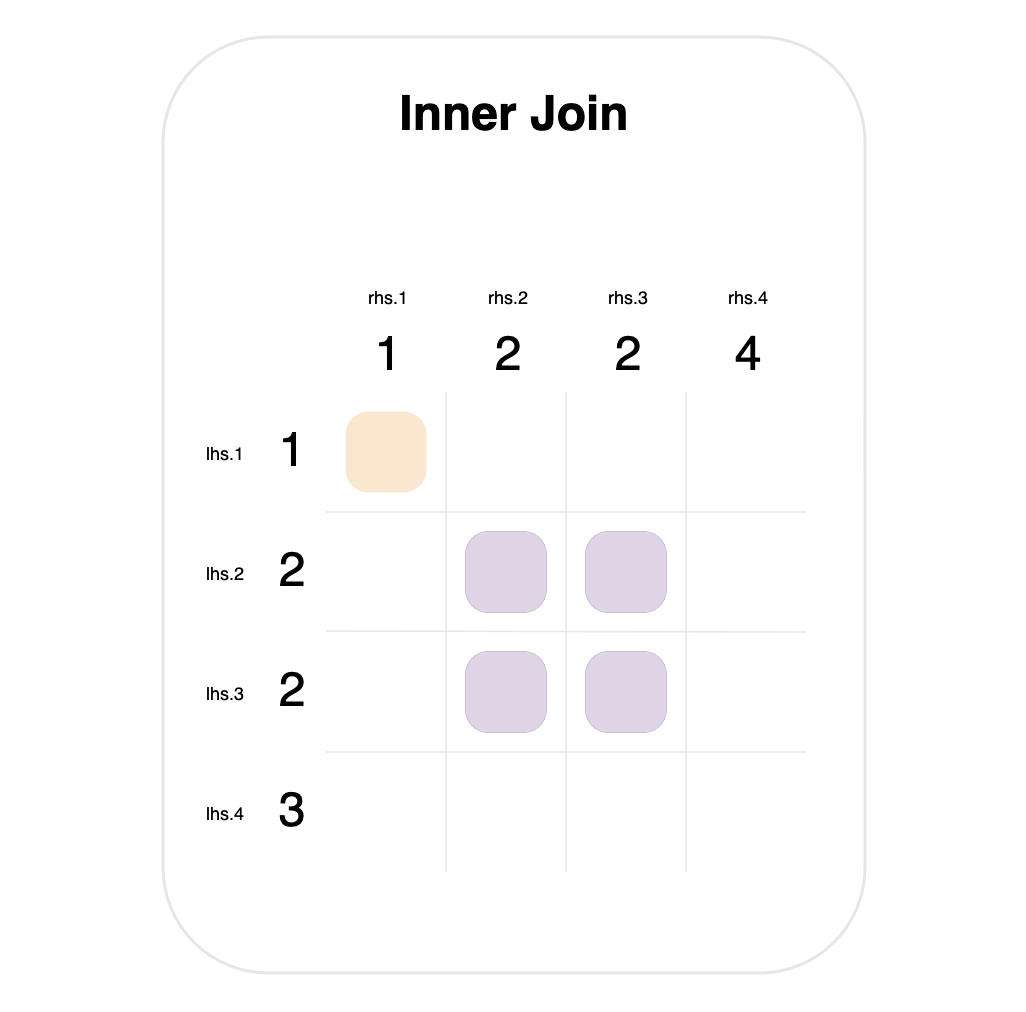This is shown in the graph above—for an inner join, where both tables contain the value `2` twice. In this case, it results in 4 matches, which will each produce a row in the final result.

Here is the code corresponding to the graph above.

``````import pandas as pd
lhs_dupes = pd.DataFrame({
"id": [1, 2, 2, 3],
"val": ["lhs.1", "lhs.2", "lhs.3", "lhs.4"]
})

rhs_dupes = pd.DataFrame({
"id": [1, 2, 2, 4],
"val": ["rhs.1", "rhs.2", "rhs.3", "rhs.4"]
})``````
``lhs_dupes``
id val
0 1 lhs.1
1 2 lhs.2
2 2 lhs.3
3 3 lhs.4
``rhs_dupes``
id val
0 1 rhs.1
1 2 rhs.2
2 2 rhs.3
3 4 rhs.4
``inner_join(lhs_dupes, rhs_dupes, on="id")``
id val_x val_y
0 1 lhs.1 rhs.1
1 2 lhs.2 rhs.2
2 2 lhs.2 rhs.3
3 2 lhs.3 rhs.2
4 2 lhs.3 rhs.3

## NA handling

By default, missing values are matched to each other in joins.

This is shown in the left join below, where each table has a single `NA` value.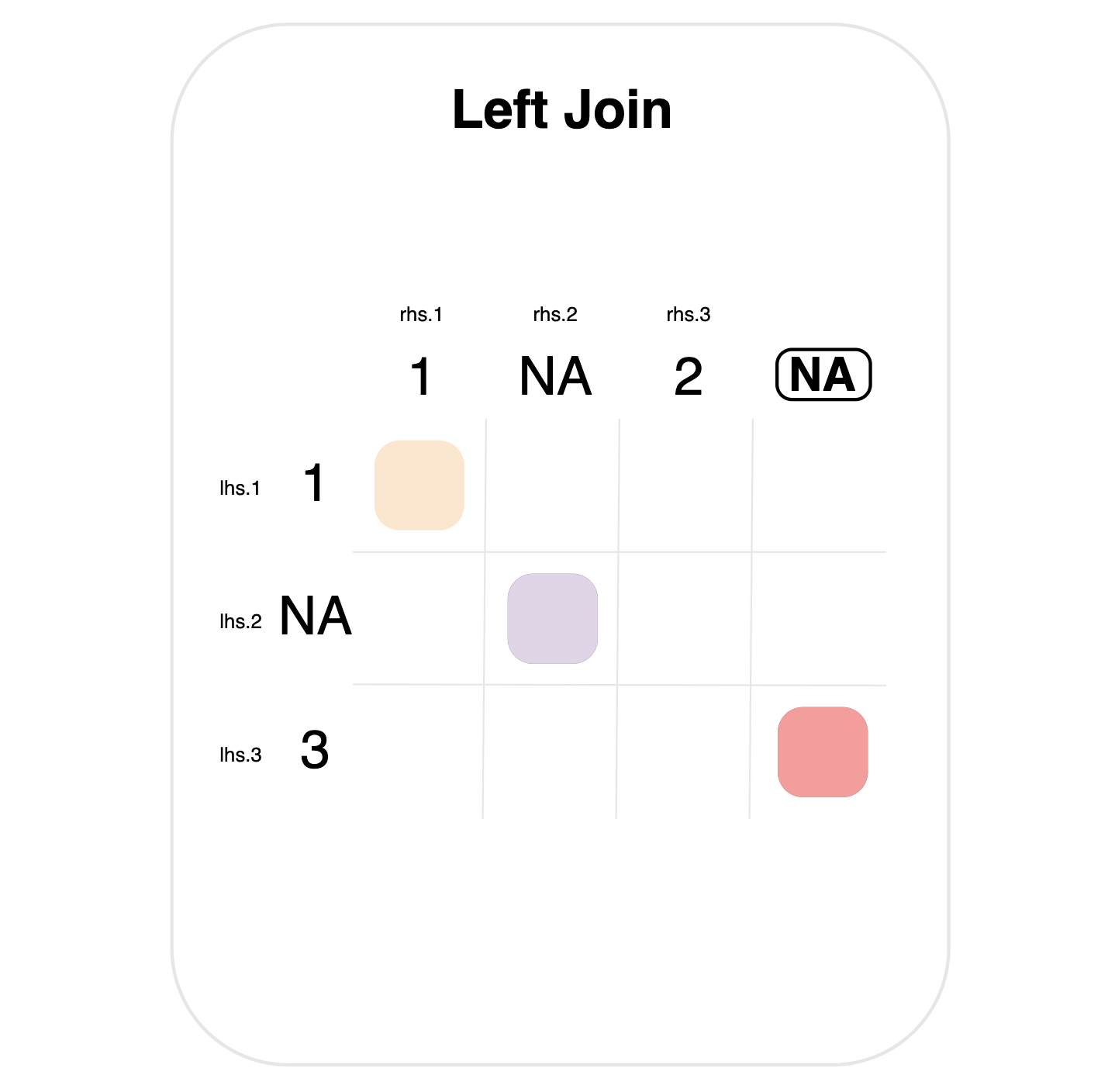Note the purple mark indicating a match between the `NA` values. Importantly, literal `NA` values here are different from the circled dummy NA (top right), which indicates the special matching rule for left joins.

Here is the code for the example:

``````import pandas as pd
lhs_na = pd.DataFrame({"id": [1, pd.NA, 3], 'val': ['lhs.1', 'lhs.2', 'lhs.3']})
rhs_na = pd.DataFrame({"id": [1, pd.NA, 2], 'val': ['rhs.1', 'rhs.2', 'rhs.3']})
left_join(lhs_na, rhs_na, on="id")``````
id val_x val_y
0 1 lhs.1 rhs.1
1 <NA> lhs.2 rhs.2
2 3 lhs.3 NaN

## Match on multiple columns

Joins can be performed by comparing multiple pairs of columns to each other— such as the `source` and `id` columns in the data below.

``````lhs_multi = pd.DataFrame({
"source": ["a", "a", "b"],
"id": [1, 2, 1],
"val": ["lhs.1", "lhs.2", "lhs.3"]
})

rhs_multi = pd.DataFrame({
"source": ["a", "b", "c"],
"id": [1, 1, 1],
"val": ["rhs.1", "rhs.2", "rhs.3"]
})``````
``lhs_multi``
source id val
0 a 1 lhs.1
1 a 2 lhs.2
2 b 1 lhs.3
``rhs_multi``
source id val
0 a 1 rhs.1
1 b 1 rhs.2
2 c 1 rhs.3

In this case, a match happens when `source` matches AND `id` matches—as shown by the composite values `(source, id)` in the graph below.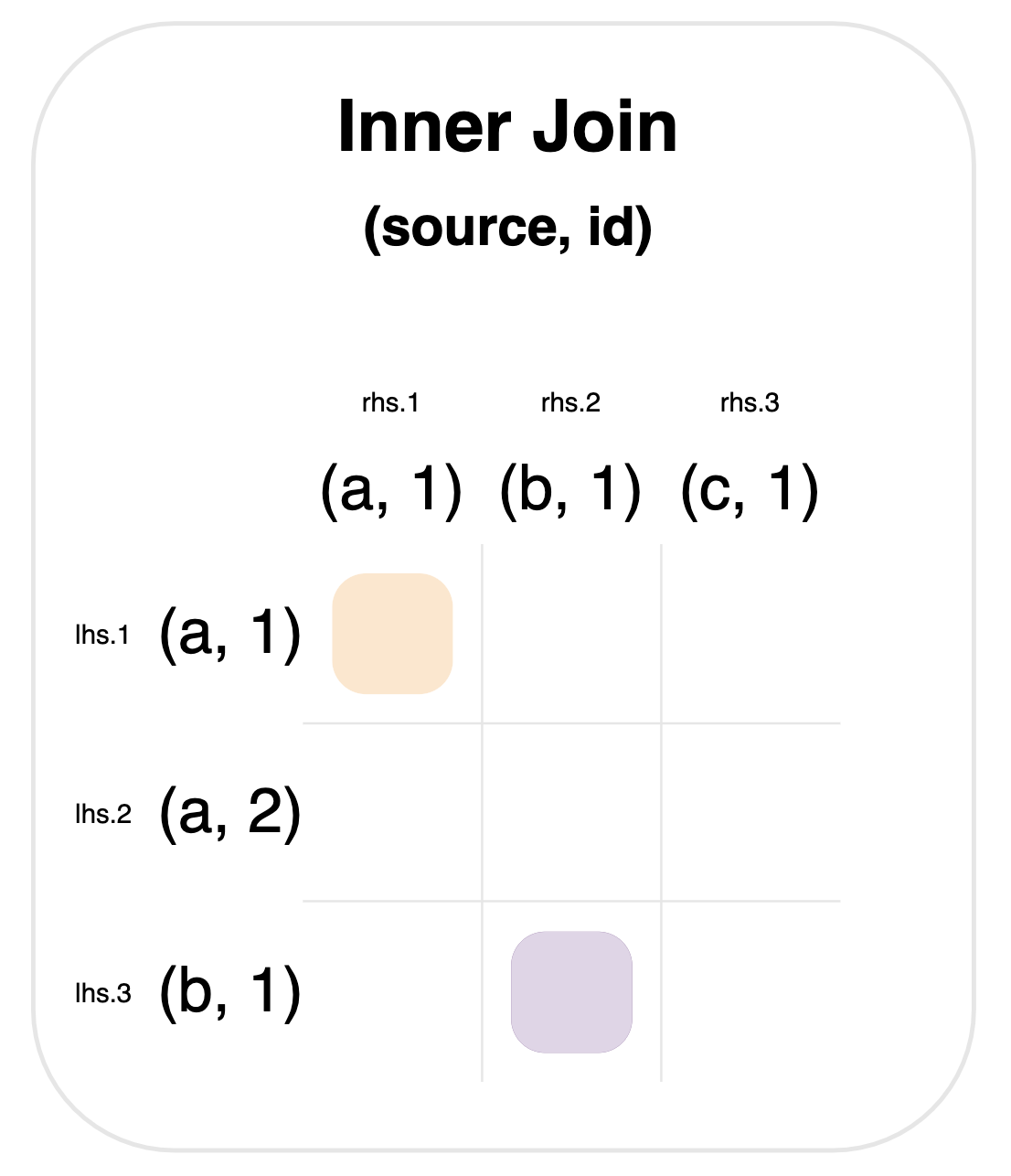### Using a list of columns

Use the `on=` argument with a list of columns, in order to join on multiple columns.

``inner_join(lhs_multi, rhs_multi, on=["source", "id"])``
source id val_x val_y
0 a 1 lhs.1 rhs.1
1 b 1 lhs.3 rhs.2

### Using a dictionary of columns

Use the `on=` argument with a dictionary to join pairs of columns with different names.

``````from siuba import rename

rhs_multi2 = rename(rhs_multi, some_source = "source")

inner_join(lhs_multi, rhs_multi2, {"source": "some_source", "id": "id"})``````
source id val_x some_source val_y
0 a 1 lhs.1 a rhs.1
1 b 1 lhs.3 b rhs.2

## Match on expressions

Some siuba backends—like those that execute SQL—can join by expressions for determining matches.

For example, the graph below shows an inner join, where a match occurs when the left-hand value is less than or equal to the right-hand value.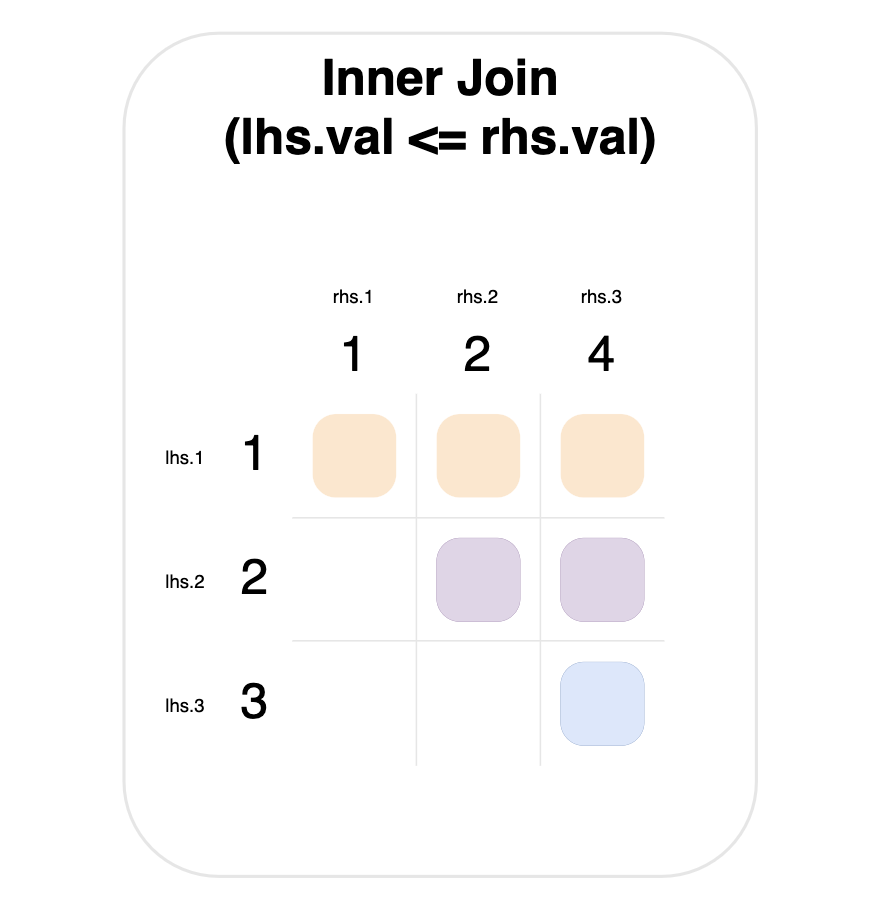Notice that the value `1` on the left-hand side matches everything on the right-hand side (top row).

### SQL backend `sql_on` argument.

Here is a full example, using sqlite:

``````from sqlalchemy import create_engine
from siuba.sql import LazyTbl

engine = create_engine("sqlite:///:memory:")

lhs = pd.DataFrame({'id': [1,2,3], 'val': ['lhs.1', 'lhs.2', 'lhs.3']})
rhs = pd.DataFrame({'id': [1,2,4], 'val': ['rhs.1', 'rhs.2', 'rhs.3']})

lhs.to_sql("lhs", engine, index=False)
rhs.to_sql("rhs", engine, index=False)

tbl_sql_lhs = LazyTbl(engine, "lhs")
tbl_sql_rhs = LazyTbl(engine, "rhs")

inner_join(
tbl_sql_lhs,
tbl_sql_rhs,
sql_on = lambda lhs, rhs: lhs.val <= rhs.val
)``````
```# Source: lazy query
# DB Conn: Engine(sqlite:///:memory:)
# Preview:
```
id_x val_x id_y val_y
0 1 lhs.1 1 rhs.1
1 1 lhs.1 2 rhs.2
2 1 lhs.1 4 rhs.3
3 2 lhs.2 1 rhs.1
4 2 lhs.2 2 rhs.2

# .. may have more rows

Note that the function passed to `sql_on` takes sqlalchemy columns for the left- and right-hand sides, so currently limited to what can be done in sqlalchemy.